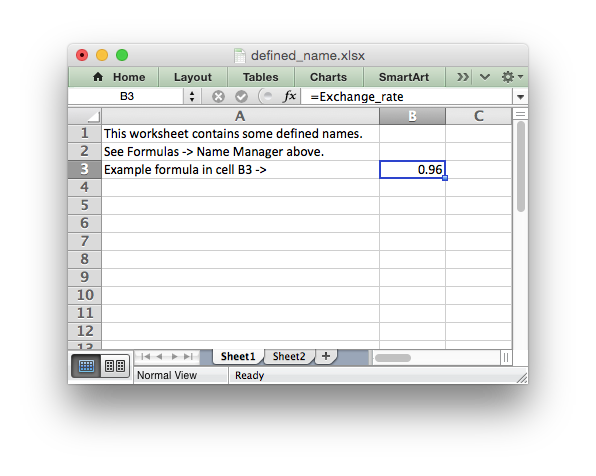libxlsxwriter
defined_name.c

Example of how to create defined names (named ranges) using libxlsxwriter.

Defined names are used to define descriptive names to represent a value, a single cell or a range of cells in a workbook or worksheet./*
* Example of how to create defined names using libxlsxwriter. This method is
* used to define a user friendly name to represent a value, a single cell or
* a range of cells in a workbook.
*
* Copyright 2014-2021, John McNamara, jmcnamara@cpan.org
*
*/
#include "xlsxwriter.h"
int main() {
lxw_workbook *workbook = workbook_new("defined_name.xlsx");
lxw_worksheet *worksheet;
/* We don't use the returned worksheets in this example and use a generic
* loop instead. */
/* Define some global/workbook names. */
workbook_define_name(workbook, "Sales", "=!G1:H10");
workbook_define_name(workbook, "Exchange_rate", "=0.96");
workbook_define_name(workbook, "Sales", "=Sheet1!\$G\$1:\$H\$10");
/* Define a local/worksheet name. */
workbook_define_name(workbook, "Sheet2!Sales", "=Sheet2!\$G\$1:\$G\$10");
/* Write some text to the worksheets and one of the defined name in a formula. */
LXW_FOREACH_WORKSHEET(worksheet, workbook){
worksheet_set_column(worksheet, 0, 0, 45, NULL);
worksheet_write_string(worksheet, 0, 0,
"This worksheet contains some defined names.", NULL);
worksheet_write_string(worksheet, 1, 0,
"See Formulas -> Name Manager above.", NULL);
worksheet_write_string(worksheet, 2, 0,
"Example formula in cell B3 ->", NULL);
worksheet_write_formula(worksheet, 2, 1, "=Exchange_rate", NULL);
}
return workbook_close(workbook);
}
workbook_close
lxw_error workbook_close(lxw_workbook *workbook)
Close the Workbook object and write the XLSX file.
worksheet_write_formula
lxw_error worksheet_write_formula(lxw_worksheet *worksheet, lxw_row_t row, lxw_col_t col, const char *formula, lxw_format *format)
Write a formula to a worksheet cell.
workbook_new
lxw_workbook * workbook_new(const char *filename)
Create a new workbook object.
LXW_FOREACH_WORKSHEET
#define LXW_FOREACH_WORKSHEET(worksheet, workbook)
Macro to loop over all the worksheets in a workbook.
Definition: workbook.h:163
lxw_worksheet
Struct to represent an Excel worksheet.
Definition: worksheet.h:2107
workbook_define_name
lxw_error workbook_define_name(lxw_workbook *workbook, const char *name, const char *formula)
Create a defined name in the workbook to use as a variable.
lxw_workbook
Struct to represent an Excel workbook.
Definition: workbook.h:280
worksheet_write_string
lxw_error worksheet_write_string(lxw_worksheet *worksheet, lxw_row_t row, lxw_col_t col, const char *string, lxw_format *format)
Write a string to a worksheet cell.
worksheet_set_column
lxw_error worksheet_set_column(lxw_worksheet *worksheet, lxw_col_t first_col, lxw_col_t last_col, double width, lxw_format *format)
Set the properties for one or more columns of cells.